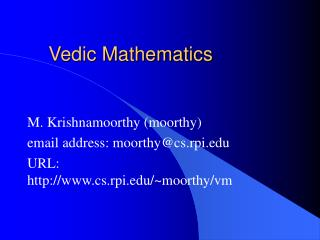Download PresentationVedic Mathematics

# Vedic Mathematics - PowerPoint PPT PresentationDownload Presentation## Vedic Mathematics

- - - - - - - - - - - - - - - - - - - - - - - - - - - E N D - - - - - - - - - - - - - - - - - - - - - - - - - - -
##### Presentation Transcript

1. Vedic Mathematics M. Krishnamoorthy (moorthy) email address: moorthy@cs.rpi.edu URL: http://www.cs.rpi.edu/~moorthy/vm

2. Sutra 1. Ekadhikena Purvena By one more than the previous one. The preposition “by” means the operations this sutra concerns are either multiplication or division ( In the case of addition/subtraction preposition to/from is used.). In this case it is useful for both of them.

3. Examples 67 * 63 72 * 78 84 * 86

4. Why does it work? x y = 10 * x + y a b = 10 * a + b x y * a b ( we know that x=a, y+b=10) (10*a + y) (10*a+b) = 100 a^2+ 10*a(y+b)+yb = 100 a*(a+1) + yb (because y+b=10)

5. Some Generalizations 23 * 37 = 46 * 74 = 22 * 68 =

6. More Uses 102 * 198 = 234 * 2 66 = 345 * 3 55 =

7. Corollary 1 for Nikhilam Yavdunam Jaavdunikritya Varga Cha Yojayet Whatever the extent of dificiency lessen it still further to that very extent; and also set up the square of that deficiency.

8. Examples Computing the square of 9: Nearest power of 10 to 9 is 10. Since 9 is 1 less than 10, decrease it 1 further to 8. Then get the square of the deficiency which is 1. Hence the answer is 81. 8^2 - deficiency is 2, 2 further of 8 is 6. 64 ( 4 is the square of 2.)

9. Examples Computing the square of 7. Nearest power of 10 to 7 is 10. Since 7 is 3 less than 10, decrease it 3 further to 4. Then get the square of the deficiency which is 9. Hence the answer is 49.. 11^2 - deficiency is 1, 1 further of 11 is 12. 121.

10. More Examples 18 ^ 2 19^2 32 ^ 2

11. Vedic Number Representation A number was encoded using a consonant groups of sanskrit alphabet, and vowels were provided as additional latitude to the author for poetic composition. The coding key is given as Kaadi nav, taadi nav, paadi panchak, yaadashtak ta ksha shunyam.

12. Meaning Translated as below: • letter “ka” and the following eight letters • letter “ta” and the following eight letters • letter “pa” and the following four letters • letter “ya” and the following seven letters • letter “ksha” for zero

13. Numbers • ka, ta, pa, ya = 1 • kha, tha, pha, ra = 2 • ga, da, ba, la = 3 • gha, dha, bha,va =4 • gna, ba,ma,sa =5 • cha,ta,sa=6 • chha,tha,sha=7

14. Numbers - Contd • Ja, da, ha =8 • jha, dha = 9 • ksha =0 Examples pa pa = 11 ma ra = 52. An interesting example of this is said to be hymn in the praise of Lord Krishna which gives the value of Pi to 32 decimal places.